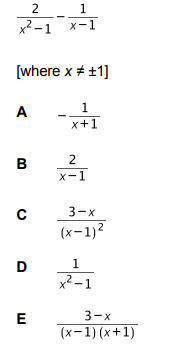# IMAT 2016 Q55 [Simplification]

Which one of the following is a simplification ofIn order to solve this question, we need to know the difference of two squares:
a^2 -b^2 = (a-b)(a+b)

\frac{2}{x^2 -1} - \frac{1}{x-1} = \frac{2}{(x-1)(x+1)} - \frac{1}{x-1} = \frac{1}{x-1}( \frac{2}{(x+1)} -1) = \frac{1}{x-1}( \frac{2 - (x+1)}{(x+1)} ) = \frac{1}{x-1} \frac{2 -x -1)}{(x+1)}=
\frac{1}{x-1} \frac{1 -x }{(x+1)} = \frac{1}{x-1} (-1)\frac{x-1}{x+1} = -\frac{1}{x+1}.

So the answer to this question is (A) -\frac{1}{x+1}.